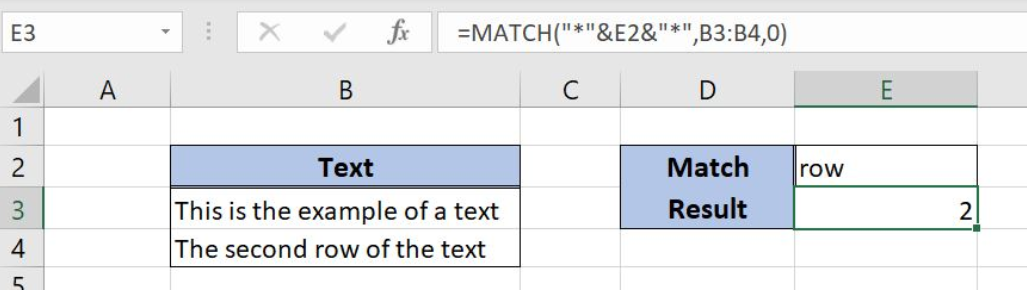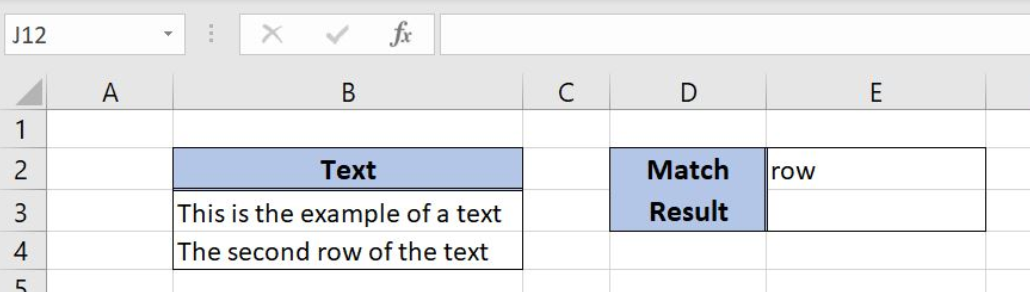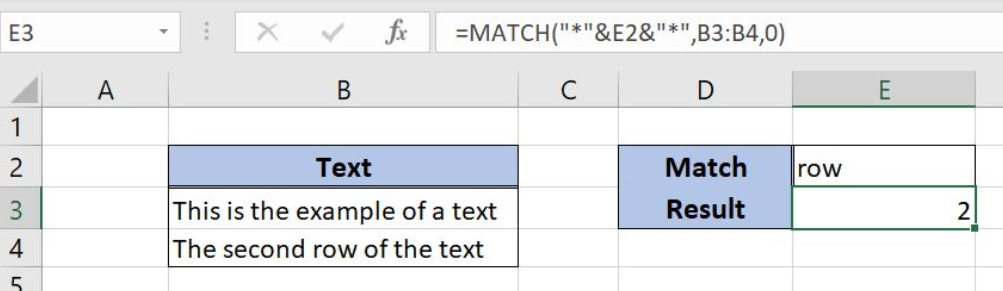Get instant live expert help with Excel or Google Sheets“My Excelchat expert helped me in less than 20 minutes, saving me what would have been 5 hours of work!”

#### Post your problem and you'll get expert help in seconds

Your message must be at least 40 characters
Our professional experts are available now. Your privacy is guaranteed.

# Position of first partial match

Excel allows us to a position of the first partial match in a range, by using the MATCH function. The function returns the first row where the selected string is founded. This step by step tutorial will assist all levels of Excel users in finding a position of the first partial match.Figure 1. The final result of the MATCH function

## Syntax of the MATCH formula

`=MATCH(lookup_value, lookup_array, [match_type])`

The parameters of the MATCH function are:

• lookup_value – a value which we want to find in the lookup_array
• lookup_array – the array where we want to find a value
• [match_type] – a type of match. We put 0 which is an exact match.

## Setting up Our Data for Finding a Position for the First Partial Match

In column B (“Text”), we have the text in two rows. In the cell E2, we have the string which position (row number) we want to find in the range B3:B4. The result of the MATCH function will be in the cell E3.Figure 2. Data that we will use in the MATCH function example

## Find a Position of the First Partial Match in a Range Using the MATCH Function

We want to get a row from the range B3:B4 in the cell E3, where the string “row” occurs for the first time.

The formula looks like:

`=MATCH("*"&E2&"*", B3:B4, 0)`

The lookup_value is “*”&E2&”*”. We add an asterisk (*) before and after E2 because we want to find text even as a part of a text. An asterisk stands for any character. The lookup_array is the range B3:B4. The match_type is 0.

To apply the MATCH function, we need to follow these steps:

• Select cell E3 and click on it
• Insert the formula: `=MATCH("*"&E2&"*", B3:B4, 0)`
• Press enterFigure 3. Using the MATCH function to find the position of a string in a text

The MATCH function will return 2, as the string “row” occurs for the first time in the second row of the range B3:B4.

Most of the time, the problem you will need to solve will be more complex than a simple application of a formula or function. If you want to save hours of research and frustration, try our live Excelchat service! Our Excel Experts are available 24/7 to answer any Excel question you may have. We guarantee a connection within 30 seconds and a customized solution within 20 minutes.

### Did this post not answer your question? Get a solution from connecting with the expert.Another blog reader asked this question today on Excelchat:## Subscribe to Excelchat.coAnother blog reader asked this question today on Excelchat: